Numerical Solution of Partial Differential Equations 446-1 Numerical Solution of Partial Differential Equations:

Finite-Difference Methods

ESAM 446-1

Fall 2000

Hermann Riecke

Convergence Tests of Variable-Step Extrapolated Runge-Kutta Scheme

In the homework a fourth-order Runge-Kutta scheme was applied to the Duffing oscillator. The initial conditions were chosen such that the particle barely made it across the barrier in the center, which makes the dynamics very sensitive to the accurace of the code.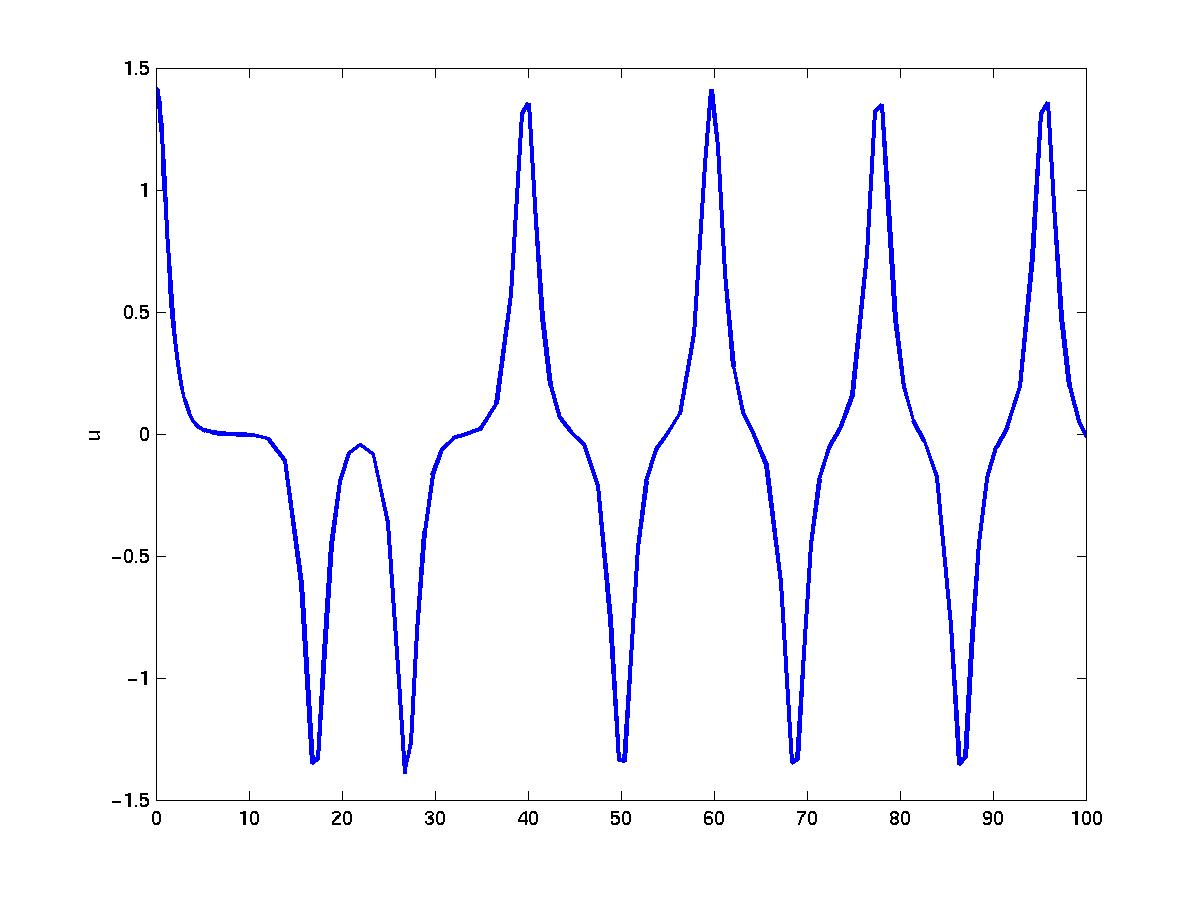Solution u for tol=0.025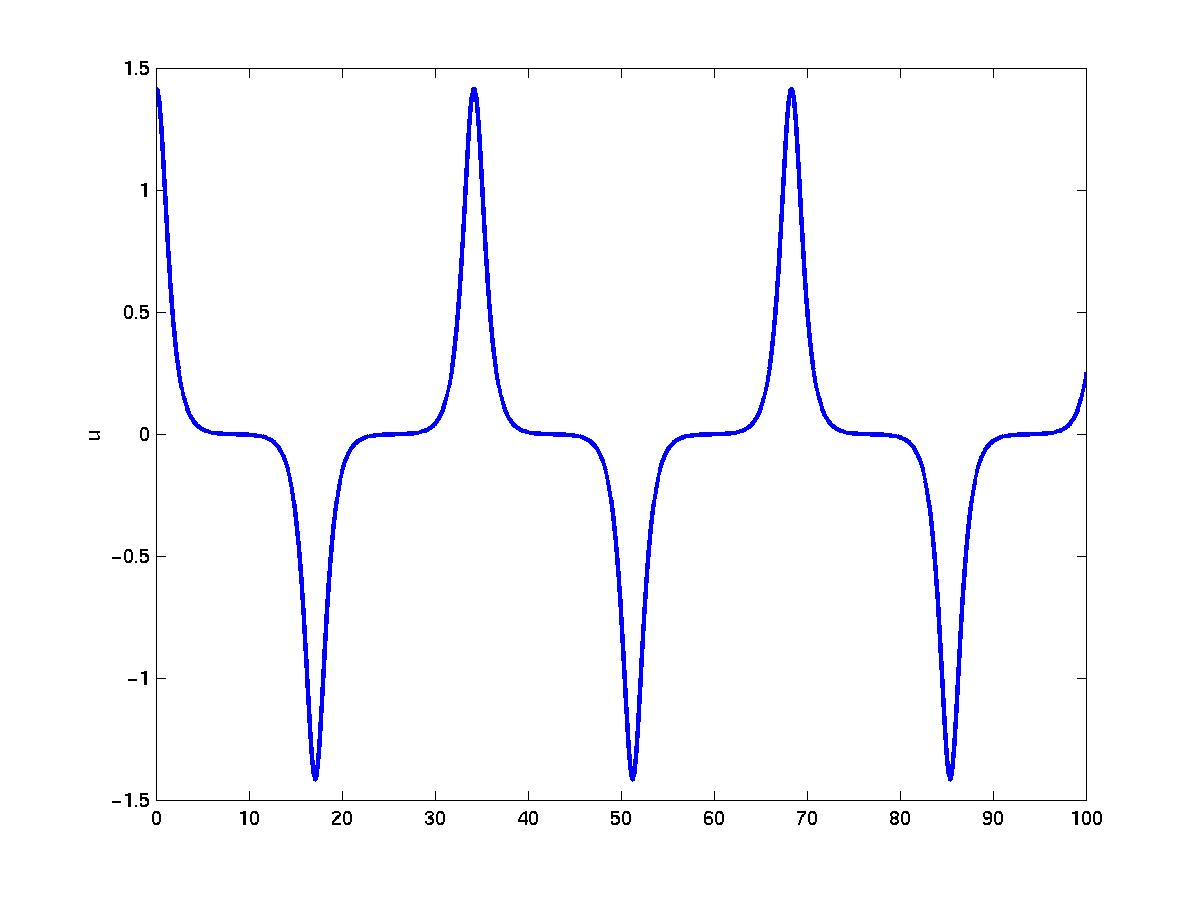Solution u for tol=1e-11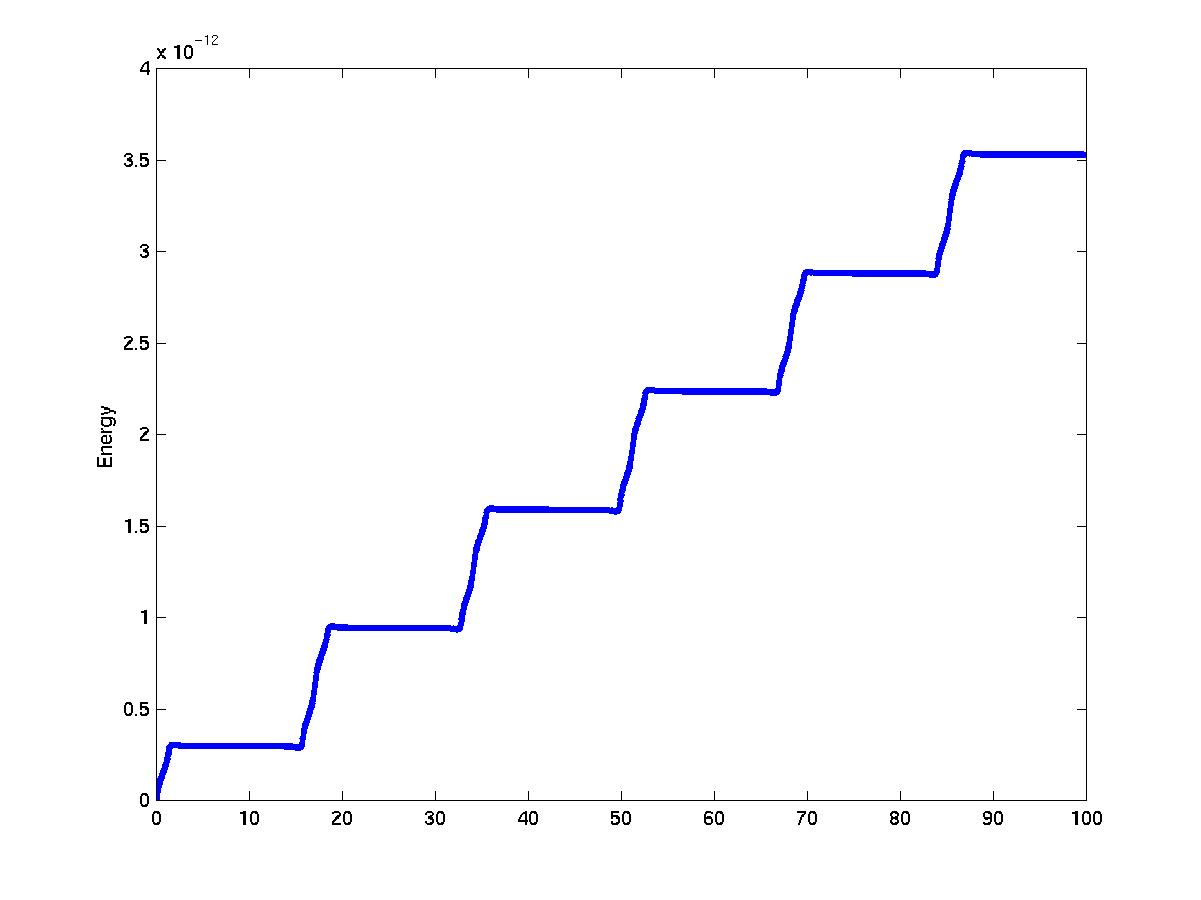Time dependence of the total energy.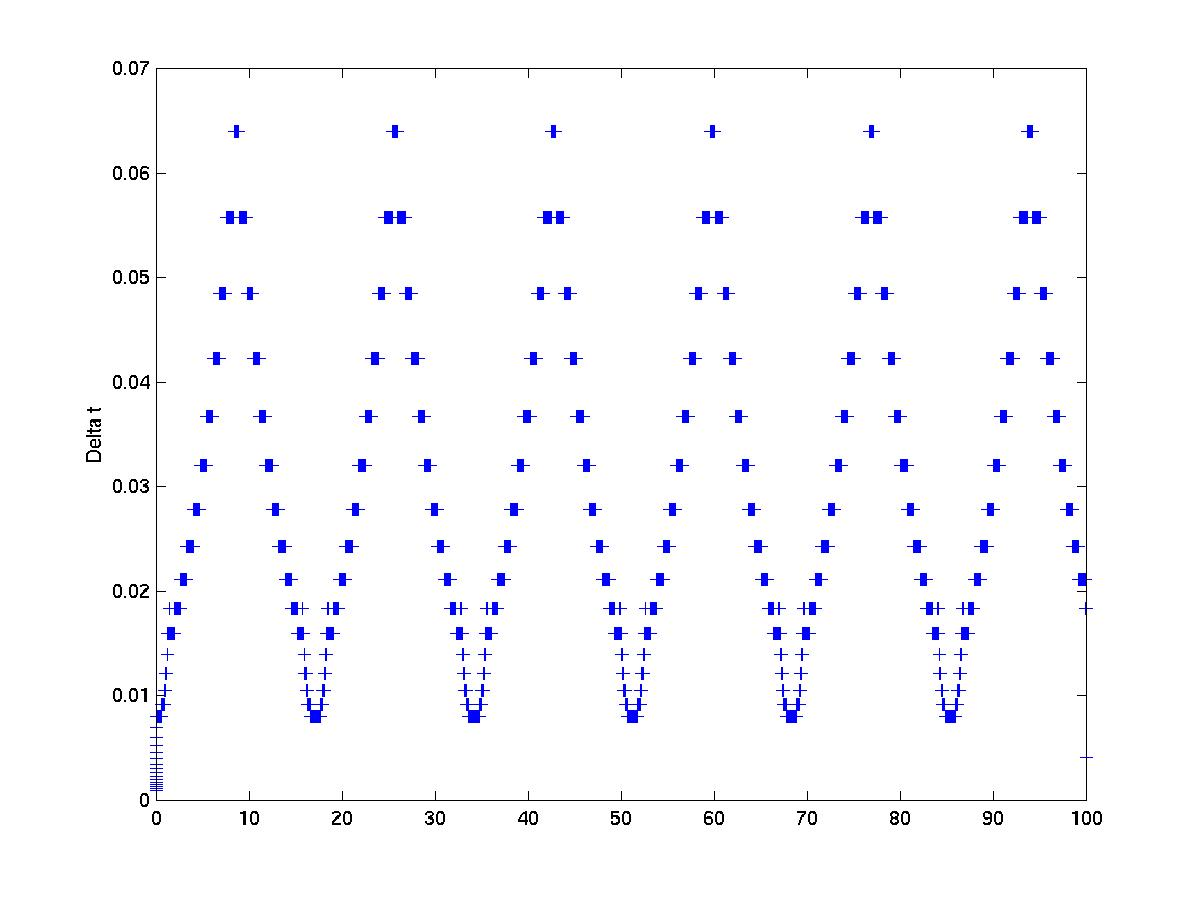Time dependence of the time steps.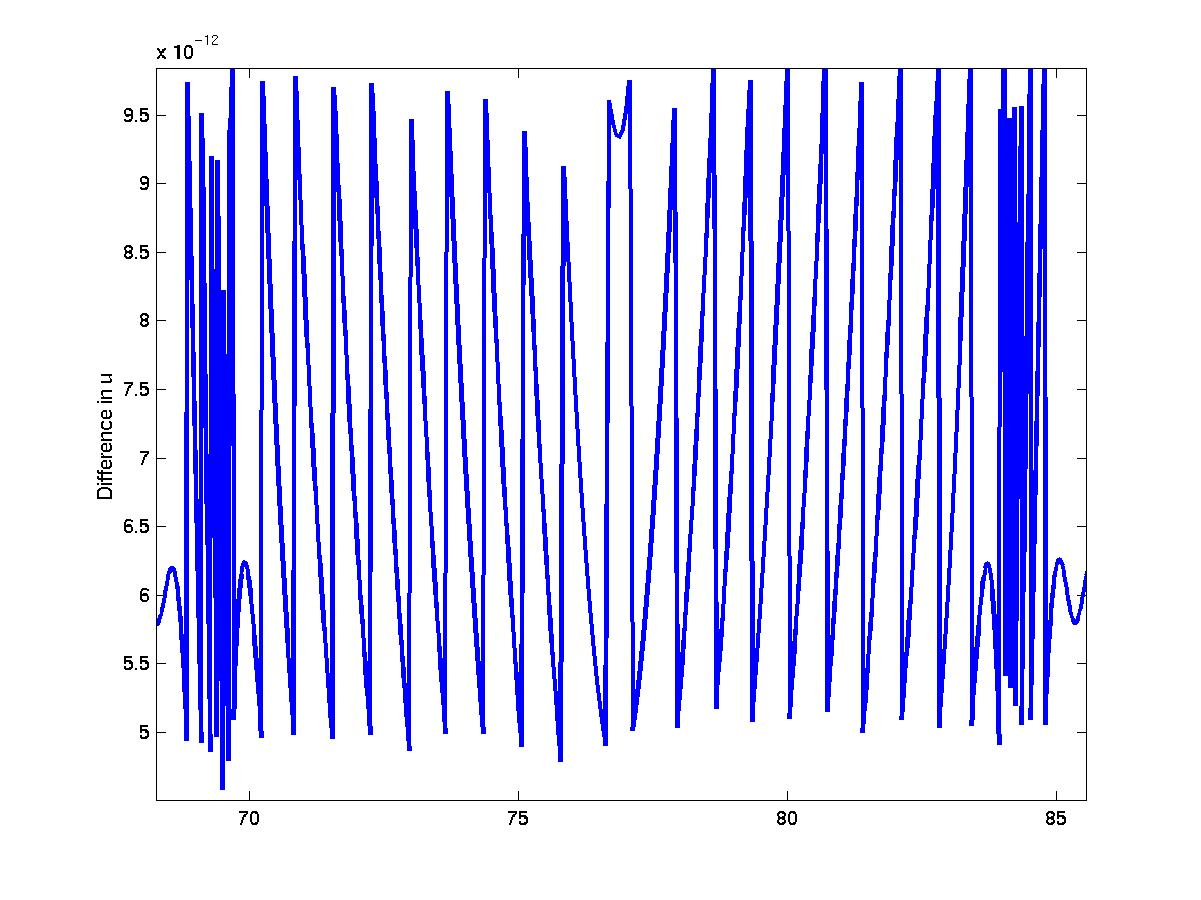Difference between approximations to u for delta t and delta t/2.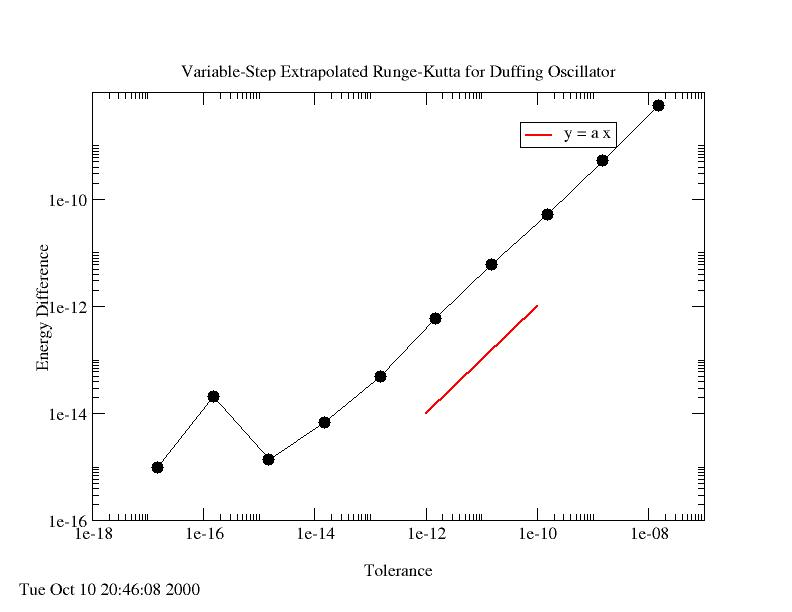Dependence of change in total energy in the oscillator on the tolerance applied in the choice of time step. The energy change and the tolerance are linearly related.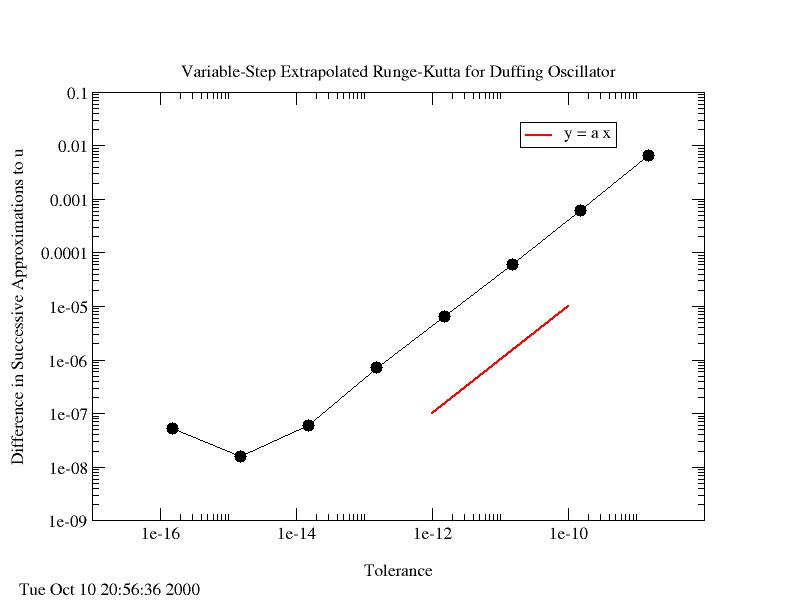The difference in the value of the dependent variable u between two approximations using timesteps delta t and delta t/2 as a function of the tolerance. The accuracy of the solution and the tolerance are linearly related.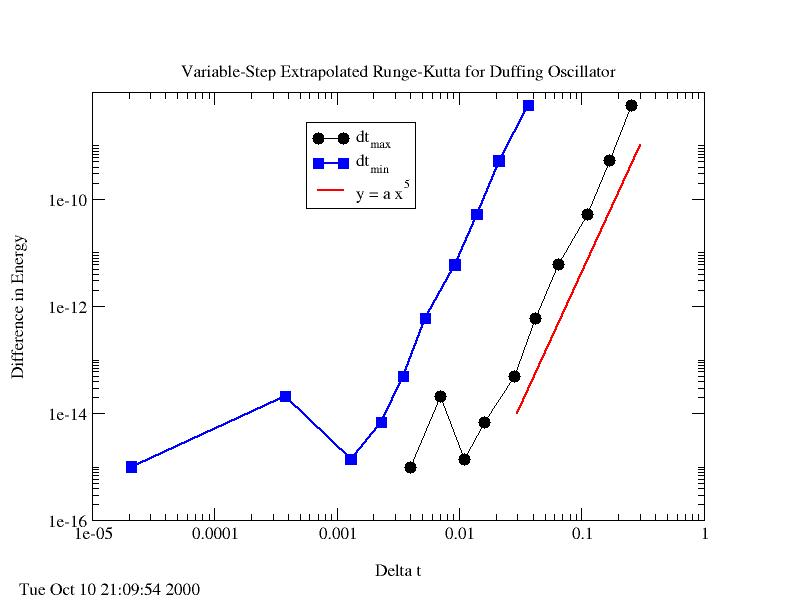The dependence of the energy change on the time step shows the 5th-order accuracy.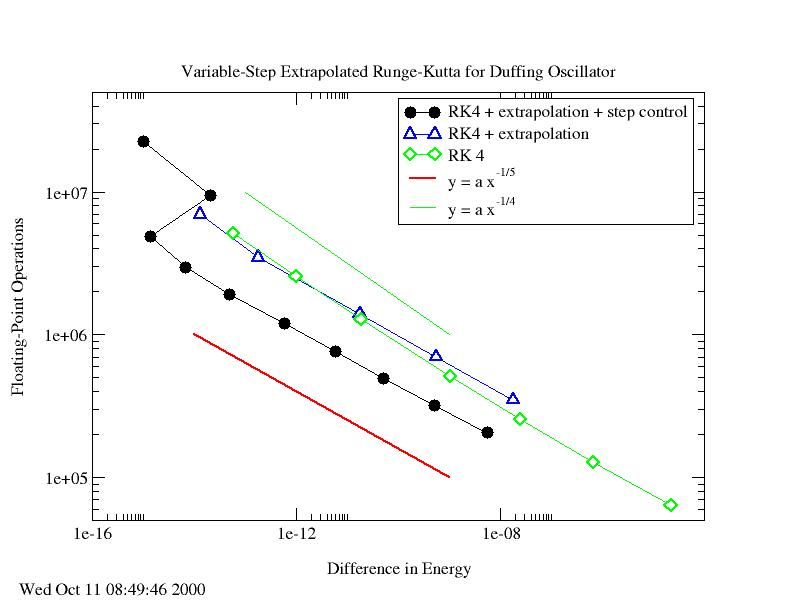The computational effort grows with the fifth-power of the accurace (change in energy).